International
Tables for
Crystallography
Volume D
Physical properties of crystals
Edited by A. Authier

International Tables for Crystallography (2013). Vol. D, ch. 3.4, pp. 517-518

Section 3.4.3.6.2. Equally deformed planes of a ferroelastic domain pair

V. Janoveca* and J. Přívratskáb

aInstitute of Physics, Academy of Sciences of the Czech Republic, Na Slovance 2, CZ-18221 Prague 8, Czech Republic, and bDepartment of Mathematics and Didactics of Mathematics, Technical University of Liberec, Hálkova 6, 461 17 Liberec 1, Czech Republic
Correspondence e-mail:  janovec@fzu.cz

3.4.3.6.2. Equally deformed planes of a ferroelastic domain pair

| top | pdf |

We start with the example of a phase transition with the symmetry descent, which generates two ferroelastic single-domain statesand(see Fig. 3.4.2.2). An `elementary cell' of the parent phase is represented in Fig. 3.4.3.5(a) by a squareand the corresponding domain state is denoted by.Figure 3.4.3.5 | top | pdf |Two ways of constructing a ferroelastic domain twin. (a) Formation of ferroelastic single-domain statesfrom the parent phase stateand then rotating away these single-domain states through an angle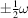about the domain-pair axis H so that disoriented ferroelastic domain statesandmeet along one of two perpendicular planes of equal deformation p or. (b) Formation of a ferroelastic twin from one ferroelastic domain stateby a simple shear deformation with a shear angle (obliquity). For more details see the text.

In the ferroic phase, the squarecan change either under spontaneous straininto a spontaneously deformed rectangular cellrepresenting a domain state, or under a spontaneous straininto rectangularrepresenting domain state. We shall use the letteras a symbol of the parent phase andas symbols of two ferroelastic single-domain states.

Let us now choose in the parent phase a vector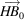. This vector changes into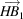in ferroelastic domain stateand into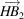in ferroelastic domain state. We see that the resulting vectorsandhave different direction but equal length: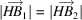. This consideration holds for any vector in the plane p, which can therefore be called an equally deformed plane (EDP). One can find that the perpendicular planeis also an equally deformed plane, but there is no other plane with this property.

The intersection of the two perpendicular equally deformed planes p andis a line called an axis of the ferroelastic domain pair(in Fig. 3.4.3.5it is a line at H perpendicular to the paper). This axis is the only line in which any vector chosen in the parent phase exhibits equal deformation and has its direction unchanged in both single-domain statesandof a ferro­elastic domain pair.

This consideration can be expressed analytically as follows (Fousek & Janovec, 1969; Sapriel, 1975). We choose in the parent phase a plane p and a unit vectorin this plane. The changes of lengths of this vector in the two ferroelastic domain statesandareand, respectively, whereandare spontaneous strains inand, respectively (see e.g. Nye, 1985). (We are using the Einstein summation convention: when a letter suffix occurs twice in the same term, summation with respect to that suffix is to be understood.) If these changes are equal, i.e. iffor any vectorin the plane p, then this plane will be an equally deformed plane. If we introduce a differential spontaneous strainthe condition (3.4.3.51) can be rewritten asThis equation describes a cone with the apex at the origin. The cone degenerates into two planes if the determinant of the differential spontaneous strain tensor equals zero,If this condition is satisfied, two solutions of (3.4.3.53) exist:These are equations of two planes p andpassing through the origin. Their normal vectors areand. It can be shown that from the equationwhich holds for the trace of the matrix, it follows that these two planes are perpendicular:The intersection of these equally deformed planes (3.4.3.53) is the axis h of the ferroelastic domain pair.

Let us illustrate the application of these results to the domain pairdepicted in Fig. 3.4.3.1(b) and discussed above. From equations (3.4.3.41) and (3.4.3.47), or (3.4.3.49) and (3.4.3.50) we find the only nonzero components of the difference strain tensor areCondition (3.4.3.54) is fulfilled and equation (3.4.3.53) isThere are two solutions of this equation:These two equally deformed planes p andhave the normal vectorsand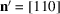. The axis h of this domain pair is directed along .

Equally deformed planes in our example have the same orientations as have the mirror planesandlost at the transition. From Fig. 3.4.3.5(a) it is clear why: reflection, which is a transposing operation of the domain pair (), ensures that the vectorsandarising fromhave equal length. A similar conclusion holds for a 180° rotation and a plane perpendicular to the corresponding twofold axis. Thus we come to two useful rules:

Any reflection through a plane that is a transposing operation of a ferroelastic domain pair ensures the existence of two planes of equal deformation: one is parallel to the corresponding mirror plane and the other one is perpendicular to this mirror plane.

Any 180° rotation that is a transposing operation of a ferroelastic domain pair ensures the existence of two equally deformed planes: one is perpendicular to the corresponding twofold axis and the other one is parallel to this axis.

A reflection in a plane or a 180° rotation generates at least one equally deformed plane with a fixed prominent crystallographic orientation independent of the magnitude of the spontaneous strain; the other perpendicular equally deformed plane may have a non-crystallographic orientation which depends on the spontaneous strain and changes with temperature. If between switching operations there are two reflections with corresponding perpendicular mirror planes, or two 180° rotations with corresponding perpendicular twofold axes, or a reflection and a 180° rotation with a corresponding twofold axis parallel to the mirror, then both perpendicular equally deformed planes have fixed crystallographic orientations. If there are no switching operations of the second order, then both perpendicular equally deformed planes may have non-crystallographic orientations, or equally deformed planes may not exist at all.

Equally deformed planes in ferroelastic–ferroelectric phases have been tabulated by Fousek (1971). Sapriel (1975) lists equations (3.4.3.55) of equally deformed planes for all ferro­elastic phases. Table 3.4.3.6contains the orientation of equally deformed planes (with further information about the walls) for representative domain pairs of all orbits of ferroelastic domain pairs. Table 3.4.3.7lists representative domain pairs of all ferroelastic orbits for which no compatible walls exist.

References

Fousek, J. (1971). Permissible domain walls in ferroelectric species. Czech. J. Phys. B, 21, 955–968.
Fousek, J. & Janovec, V. (1969). The orientation of domain walls in twinned ferroelectric crystals. J. Appl. Phys. 40, 135–142.
Nye, J. F. (1985). Physical Properties of Crystals. Oxford: Clarendon Press.
Sapriel, J. (1975). Domain-wall orientations in ferroelastics. Phys. Rev. B, 12, 5128–5140.